# GMAT Math : Acute / Obtuse Triangles

## Example Questions

1 2 3 4 5 7 Next →

### Example Question #10 : Calculating The Height Of An Acute / Obtuse Triangle

Given: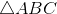with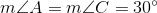and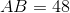.

Construct the altitude offromto a pointon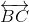. What is the length of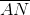?

Possible Answers: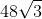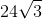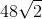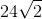Correct answer:Explanation:is shown below, along with altitude; note that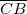has been extended to a ray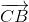to facilitate the location of the point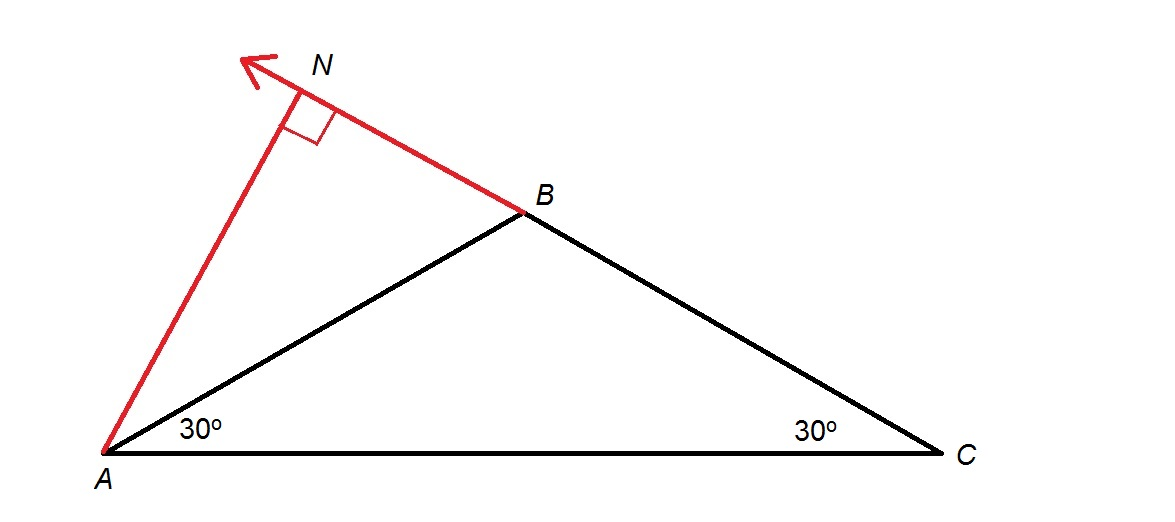Since an exterior angle of a triangle has as its measure the sum of those of its remote interior angles,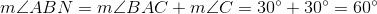By definition of an altitude,is perpendicular to, making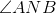a right triangle and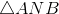a 30-60-90 triangle. By the 30-60-90 Triangle Theorem, shorter leg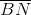ofhas half the length of hypotenuse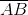—that is, half of 48, or 24; longer leghas length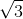times this, or, which is the correct choice.

### Example Question #61 : Acute / Obtuse Triangles

Given:withand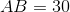.

Construct the altitude offromto a pointon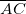. What is the length of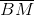?

Possible Answers: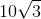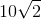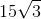Correct answer:Explanation:is shown below, along with altitude.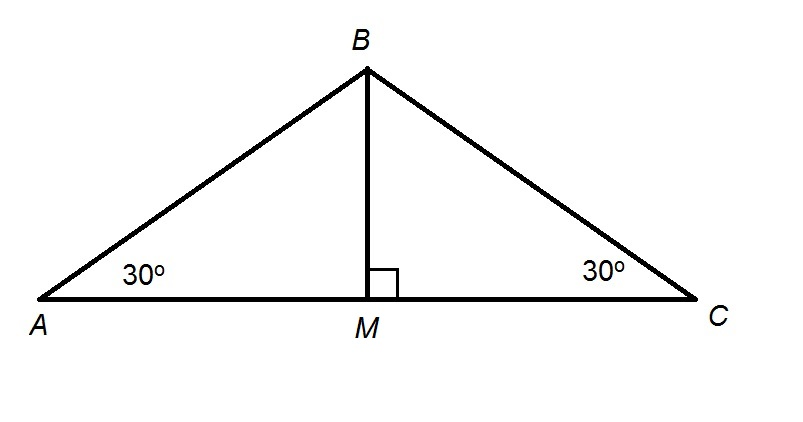Since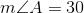, and, by definition, is perpendicular to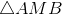is a 30-60-90 triangle. By the 30-60-90 Triangle Theorem,, as the shorter leg of, has half the length of hypotenuse; this is half of 30, or 15.

### Example Question #62 : Acute / Obtuse Triangles

Given:with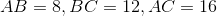, construct two  altitudes of: one fromto a pointon, and another fromto a pointon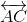. Which of the following is true of the relationship of the lengths of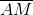and?

Possible Answers:

The length ofis nine-sixteenths that ofThe length ofis three-fourths that ofThe length ofis two-thirds that ofThe length ofis four-ninths that ofThe length ofis twice that ofCorrect answer:

The length ofis three-fourths that ofExplanation:

The area of a triangle is one half the product of the length of any base and its corresponding height; this is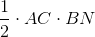, but it is also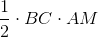. Set these equal, and note the following: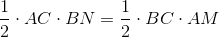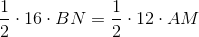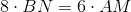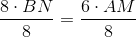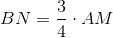That is, the length ofis three fourths that of that of1 2 3 4 5 7 Next →

### All GMAT Math Resources